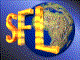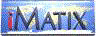| iMatix home page | << | < | > | >>SFLVersion 2.10

### csecs_to_time

```#include "sfldate.h"
long
csecs_to_time (long csecs)
```

#### Synopsis

Converts a number of centiseconds (< INTERVAL_DAY) into a time value (HHMMSSCC).

#### Source Code - (sfldate.c)

```{
long
hour,
min,
sec;

ASSERT (csecs < INTERVAL_DAY);

hour  = csecs / INTERVAL_HOUR;
csecs = csecs % INTERVAL_HOUR;
min   = csecs / INTERVAL_MIN;
csecs = csecs % INTERVAL_MIN;
sec   = csecs / INTERVAL_SEC;
csecs = csecs % INTERVAL_SEC;
return (MAKE_TIME (hour, min, sec, csecs));
}
```

 | << | < | > | >>Copyright © 1996-99 iMatix Corporation